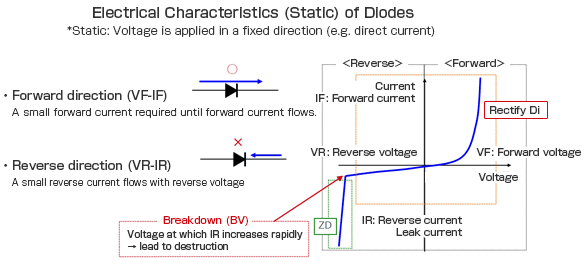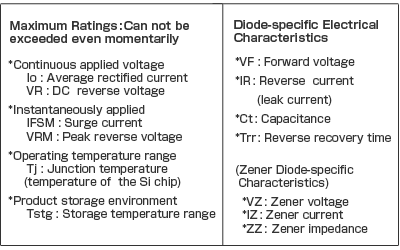# Characteristics of a Diode | What are diodes | Properties and Characteristics

## Characteristics of a Diode | What are diodes | Properties and Characteristics

### Diode Characteristics

A diode is simply a PN junction, but its applications are extensive in electronic circuits.  Three important characteristics of a diode are, first of all, the forward voltage drop.  Under a forward bias condition, this should be about .7 volts.  Then there is the reverse voltage drop.  In the reverse, when we reverse bias the diode the depletion layer widens and usually, the applied voltages are felt across the diode.  Then there is the reverse breakdown voltage.  Reverse voltage drop that will reverse current flow and in most cases destroy the diode.

One has to study characteristics of a diode in forward bias as well as in reverse bias

The forward bias characteristics of a diode is in first quadrant while reverse bizs characteristic is in third quadrant.

As seen from the characteristics, initially the current is very small for the first few tenths of a volt of applied voltage. As we reach the barrier potential, large number of electrons and holes gain enough energy to cross the junction and the current builds up rapidly. Thus above this voltage, very small increase in external voltage, produces a large change in current.The voltage at  whats the current starts increasing very rapidly, is known as the 'Knee voltage" or "offset voltage'. For Si diode this voltage is 0.7 V while for Ge diode it is 0.2 V. This difference is because of different band gap energies of two crystal structures.

As stated earlier, the reverse current of the diode is very less. The current is called reverse saturation current which is because of minority carriers. If one continues increasing the external reverse voltage, the breakdown voltage of the diode is reached. Once the breakdown reaches, a large number of the minority carriers suddenly appear in the depletion layer and the diode conducts heavily. T

he diode reverse current suddenly increases while the reverse voltage remains breakdown voltage. Ultimately diode breaks down if current is not controlled. The breakdown voltage of a particular diode is given in the data sheet.Characteristics of a Diode